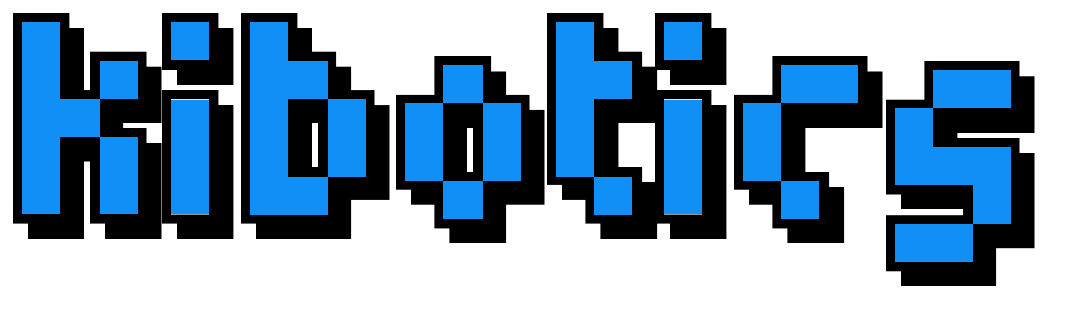# Follow line with F1 in Python

 Study time Difficulty 3 hours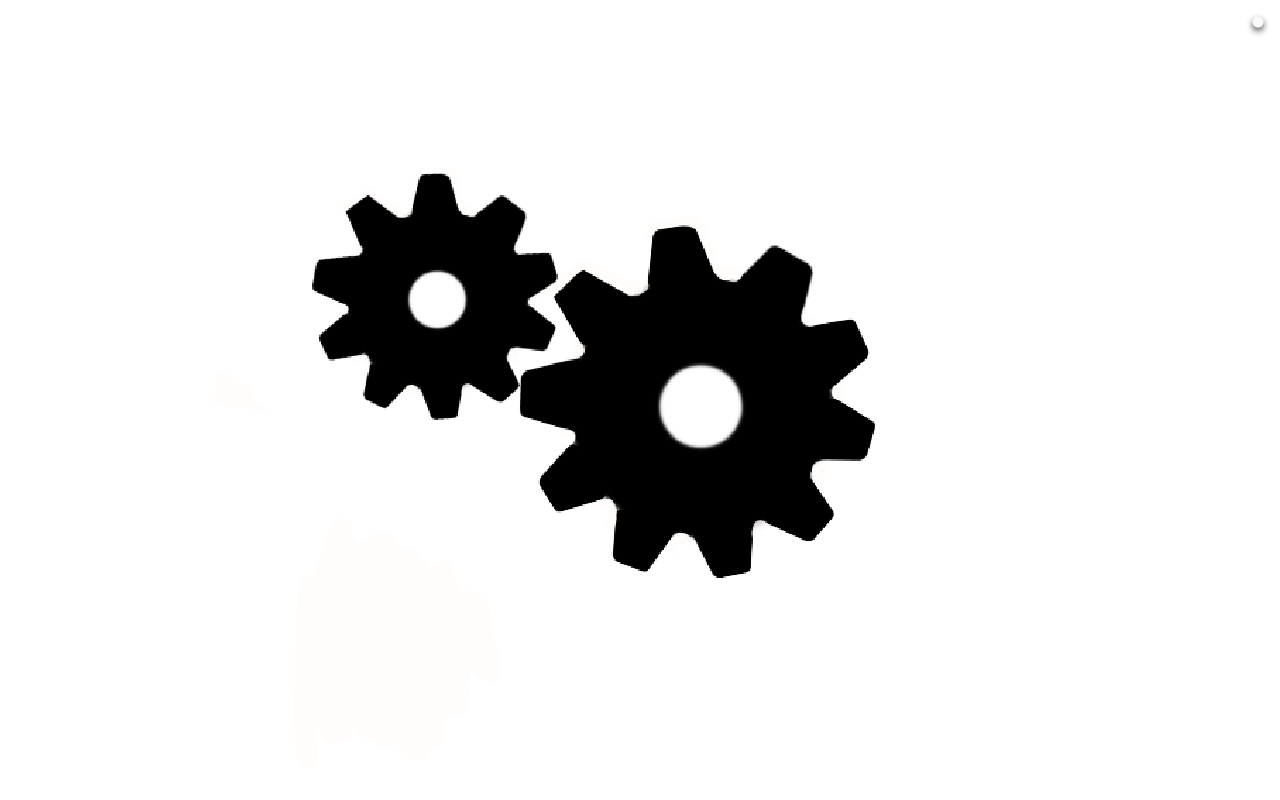In this practice you will have to programme a simulated Formula 1 car to follow the line of a circuit using the images from your camera.

Using the camera's API, you will have to turn the Formula 1 to correct the steering and get it to complete the circuit. Can you do it?

To complete this exercise, you will have to get the Formula 1 to follow the white line of the road through its vision sensor, i.e. using the images it collects with its camera.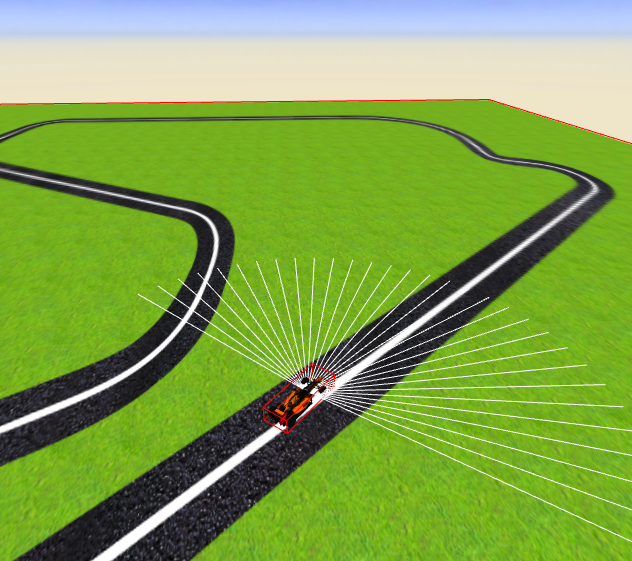## 1 - What you will learn in this unit

In this unit you are going to work with artificial vision, specifically you are going to use the camera of the simulated formula one robot to detect the white line we want to follow.

You will also learn how a digital image is represented on the computer and what the RGB colour space is

You may already have some previous experience with the use of vision in Kibotics. In this unit we will delve a little deeper into machine vision.

## 2 - Practice requirements.

You are asked to implement an algorithm, in the indicated area, that allows the Formula 1 robot to follow the path marked by the white line on the road autonomously, using the code blocks available in the work area.

But... How can we detect a line in an image?

The simplest way is to use one of the most important properties of RGB images, colour. We will detect the white line by filtering this colour in the images, so that white is the only colour that the robot "sees". In this way, it will be much easier to follow the line, without other elements interfering with the task.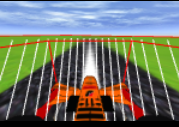## 3 - Digital images

The first question we have to ask ourselves when we start working with computer vision is how an image is represented on a computer, or in other words what a digital image is.

In order to work with images, the first thing we need is a way to represent those images in a digital medium, so we have to find a way to transform an image into a collection of numbers. To do this, what we do is to "grid" the image: divide it into a certain number of squares. Each of these squares is called a pixel. The more pixels our image has, the more similar it will be to a non-digital image.

Each of these pixels will have a colour, so we also have to "invent" a way to code these colours, i.e. to assign each colour a number. Our first idea could be just that, we give each colour a different number, like in colour painting games. For example, a 1 for red, a 2 for yellow, a 3 for green... The problem with this simple coding is that if we want to represent a wide range of colours we need a number for each colour and a huge table to determine which number corresponds to that particular colour. Another, not lesser, problem is to know exactly what the colour is. This problem is solved with RGB encoding.

### 3.1- RGB

RGB images represent each colour by combining the three primary colours red, green and blue. In this way, each pixel will have 3 numbers associated with it, one for each of the primary colours. This is why it is said to be an additive colour model, because each colour is represented by the sum of the three primary light colours.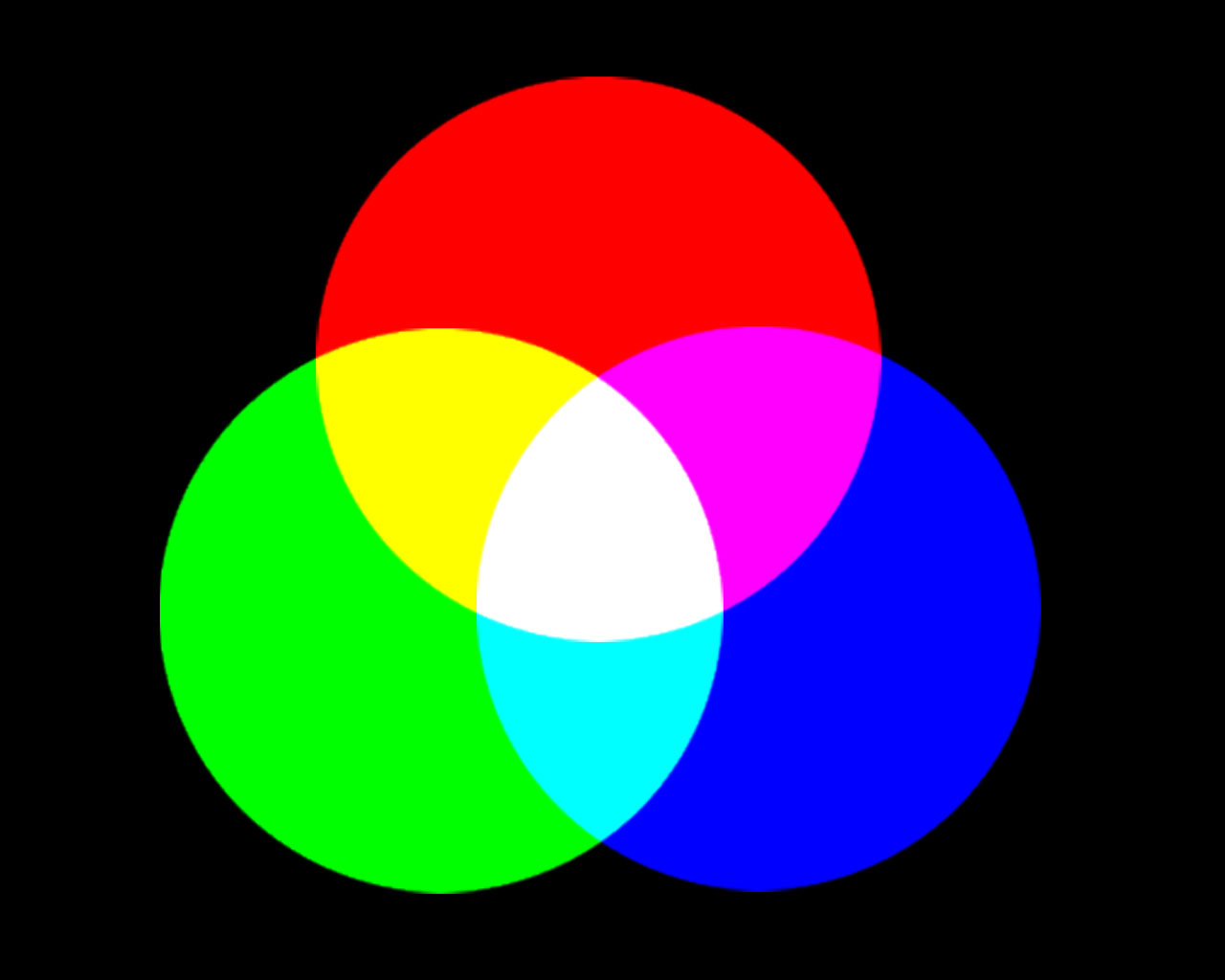A major drawback of the RGB model is that it does not detail which colour it considers red, green or blue. Therefore, the same RGB number can display noticeably different colours depending on the colour model of the device.

#### How do we use RGB?

To determine (on a computer) a colour in RGB we simply have to give 3 numbers between 0 and 255. For example (255,0,0) in this way what we are saying is that our pixel has a colour that is the sum of the maximum red component and no green or blue components. The absence of the three components would give us the colour black, which would be represented as (0,0,0). On the other hand, the white colour will be the sum of the maximum components of all the primary colours, i.e. (255,255,255)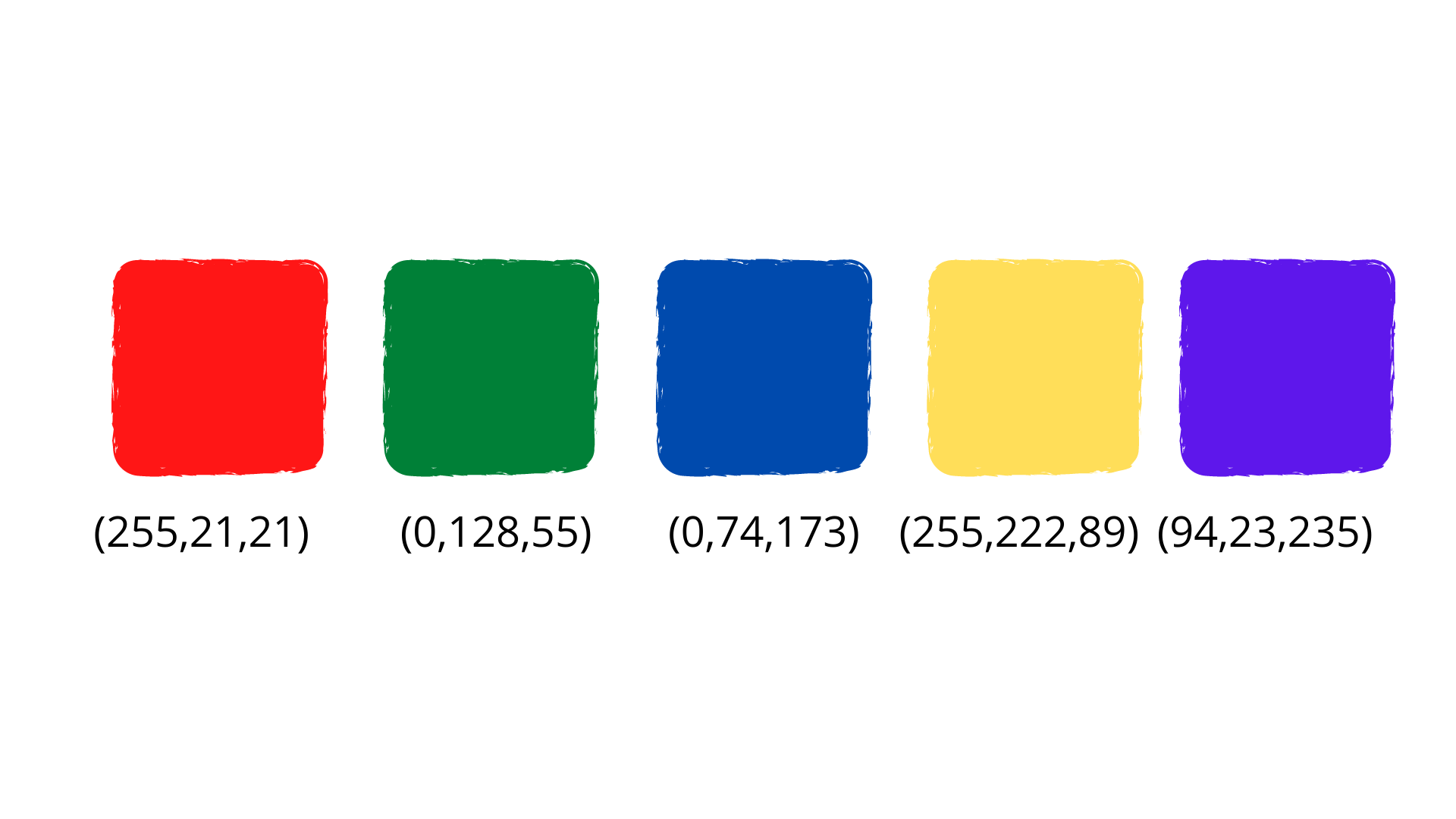## 4 - Hints for programming this exercise

Here are some tips on how to start programming this exercise.

### 4.1- Initialisation

To start programming your robot, you must first import the access module into the hardware layer of your robot. Include this code block in your editor:

`import HAL#enter your robot code here`

Remember that your code can be executed sequentially (instructions that are only executed once), or iteratively (code that is executed in a loop). Below is an example of how to use instructions of each type:

`import HAL#THESE ARE SEQUENTIAL INSTRUCTIONSHAL.advance_to(3) # the robot advances 3 metres and stopswhile (True): # THESE ARE ITERATIVE INSTRUCTIONS HAL.turn_right_to(10) # the robot turns 10 degrees to the right in each iteration, it never stops`

### 4.2- Robot Sensor and Actuator API

The functions and methods you can use to get information from the robot and send it commands to solve the practice are as follows:

#### Sensors

As we said at the beginning, with this exercise we are going to learn how to use the camera to make our simulated formula 1 follow a white line. You will need to use the camera functions, specifically the one that allows you to obtain the desired coordinate of an object of a certain colour. Think carefully about what colour you want to detect and also the coordinate(s) you will need to "drive" the formula 1 following the white line. Here are some of the functions that you may find useful.

• `get_object_color(color)`: Returns a python dictionary with two keys: 'areas' which returns the number of found objects of that colour and 'details' which is a list of dictionaries (one dictionary for each found object) with the following keys:

• 'area' showing the area of the object
• 'centre' which is a tuple with the x and y coordinates of the centre
• 'corner1' which is a tuple with the x and y coordinates of the upper right corner of an imaginary rectangle that will surround the object.
• 'corner2' which is a tuple with the x and y coordinates of the lower left corner of an imaginary rectangle surrounding the object.
• `read_us()`: This method allows you to access the robot's ultrasonic (US) sensor. Use it to obtain a distance value to the nearest object, if available.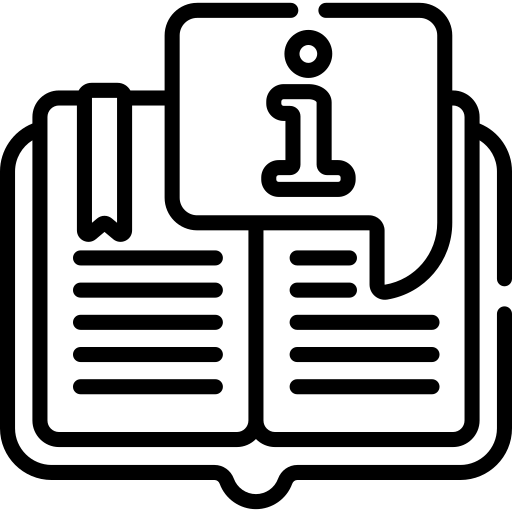More information: Camera sensor pill

#### Actuators

Once you know where your formula 1 needs to move to follow the white line, you will need to send it the correct instructions to do so. You can use the commands that send distances or turns or those that indicate speeds.

• `advance_to(distance)`: To advance a specific distance (m).

• `turn_right_to(angle)`: To turn right by a specific angle (degrees).

• `turn_left_to(angle)`: To turn left by a specific angle (degrees).

• `advance(speed)`: Indicates the linear (forward) speed of the robot (m/s).

• `turn_right(speed)`: Indicates the angular (rotational) speed of the robot (degrees/s).

• `turn_left(speed)`: Indicates the angular (rotational) speed of the robot (degrees/s).

## 5 - Compiling

This exercise is not a simple challenge, so by solving it you will have learned a lot:

1. You will have learned how digital images are represented and what a pixel is.
2. You will also have learned how colours are represented using RGB.
3. You will have used the Kibotics instruction to get the position of an object of one colour in the image.
4. You will have controlled the robot to adjust its speed and/or rotation according to the position of the white line on its front camera.

## 6 - Did you know that...?

The RGB model is not the only one used to represent colour. There are other possibilities. One of them is the so-called CMYK model (Cyan, Magenta, Yellow, Black)

The CMYK model is a subtractive model because each colour is constructed as the amount of the other colours that must be subtracted from white to obtain the desired colour. Remember that RGB is an additive model because each colour is constructed as the sum of the primary colours red, green and blue.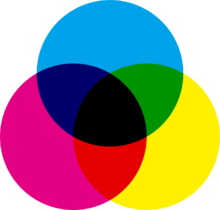It is a very suitable model for printing on white paper. The colour that remains is the subtraction of the colours of the inks on the white of the canvas. Unlike RGB, if we now add the 3 basic colours (which in this model are cyan, magenta and yellow) what we get is black.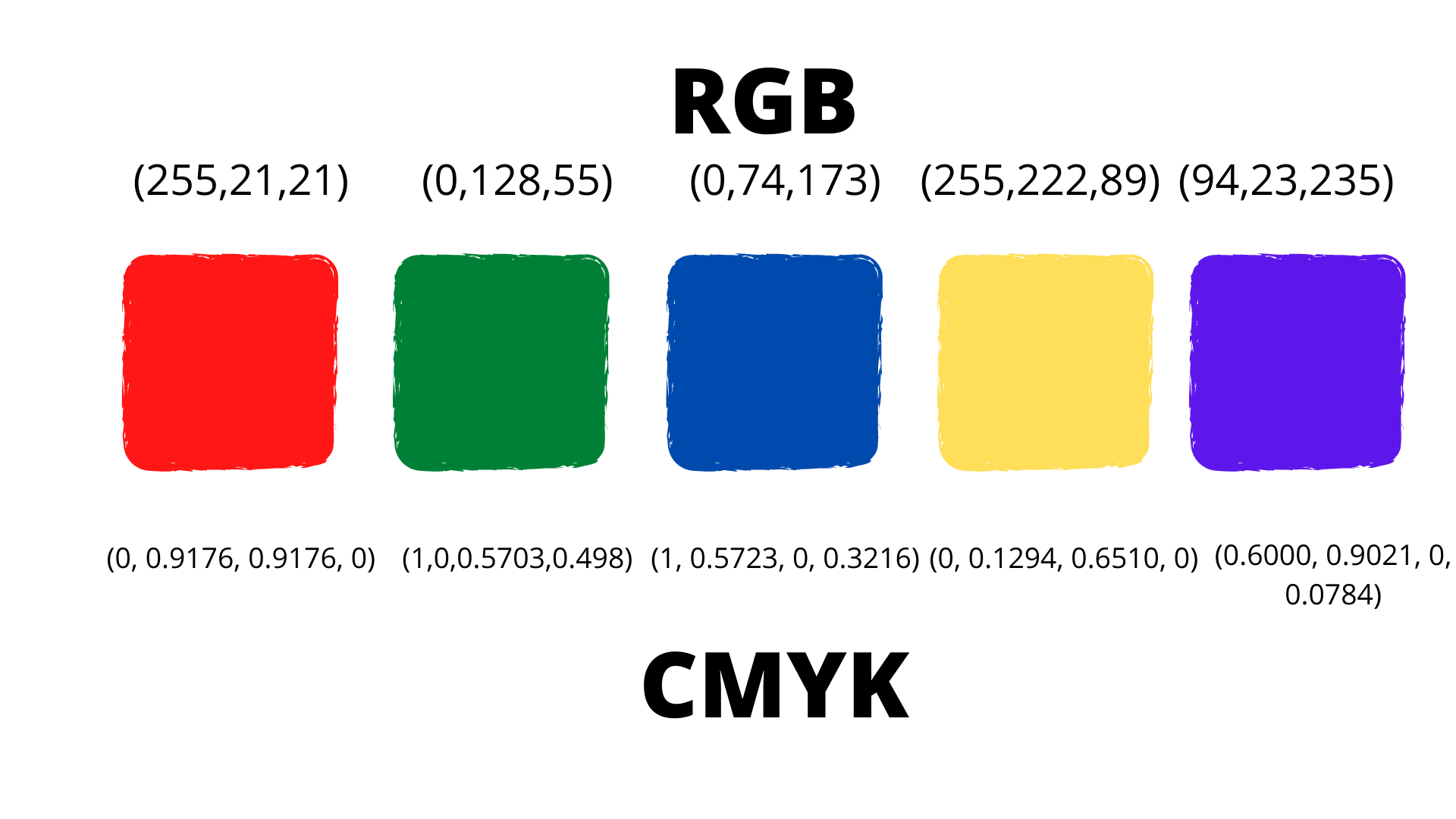## 7- Example video0%
Tiempo: 00:00
0